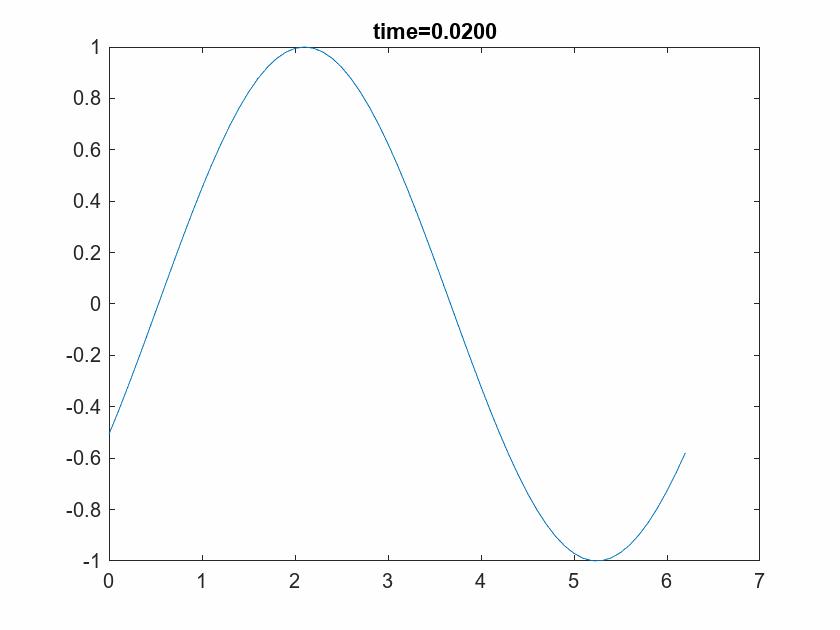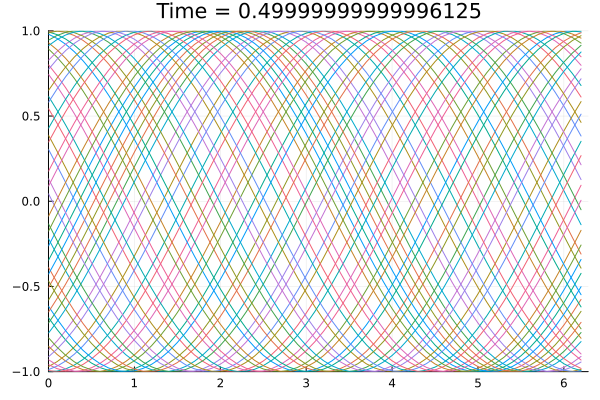# How to create a gif file of plot?

I’m a new user of JULIA. I’m trying to line by line translation of the following MATLAB code

``````t=0;
dt =1e-4;
tmax=0.5;
nmax=round(tmax/dt);

for n=1:nmax
t=t+dt;
if mod(n,100)==0
x = 0:0.1:2*pi;
y = sin(x + (n/2));
plot(x, y)
title(sprintf('time=%1.4f',t))
pause(0.1)
end
end
``````

which gives the following outputMy attempt for JULIA code is as follow:

``````using Plots
t=0
dt =1e-4;
tmax=0.5;
nmax=round(tmax/dt);
p=plot()
for n=1:nmax
t=t+dt
if mod(n,100)==0
x = 0:0.1:2π
y = sin.(x .+ (n/2))
plot!(x, y, xlims=(0,2π), ylims=(-1,1),legend=false,fmt=png)
title!("Time = \$t")
sleep(0.1)
end
end
p
``````

which givesI want to have a gif file like MATLAB with same code.

1 Like
``````ENV["GKS_WSTYPE"] = "mp4"

using GR

function main()
t = 0
dt = 1e-4
tmax = 0.5
nmax = round(tmax / dt)
for n = 1:nmax
t = t + dt
if mod(n, 100) == 0
x = 0:0.1:2π
y = sin.(x .+ (n / 2))
plot(x, y, xlim=(0,2π), ylim=(-1,1), title="Time = \$t", grid=false)
end
end
end

main()
``````
1 Like

The above in Julia REFL giving

not a movie.

Also in Jupyter notebook its not giving any output.

Did you set the environment before importing GR?

`ENV["GKS_WSTYPE"] = "mp4"`

What you probably want to do is this:

``````using Plots
t=0
dt =1e-4;
tmax=0.5;
nmax=round(tmax/dt);
anim = Animation()
for n=1:nmax
t=t+dt
if mod(n,100)==0
x = 0:0.1:2π
y = sin.(x .+ (n/2))
plot(x, y, xlims=(0,2π), ylims=(-1,1),legend=false,title="Time = \$t")
frame(anim)
end
end
gif(anim, "lol.gif", fps=10)
``````

In short, you need to create a special Animation object and load the frames into it. Refer to this manual for more info.

The documentation for creating videos in make can be found [here](Animations · Makie, where I have linked to the section that uses `Observables` to animate a wave with changing time. It should be simple to change the code so that it is the frequency that changes.
Note also that often, creating the video file can take a long time. If I only want to see an animation, and do not need to share the file, I generally love to change the value of an observable in a loop, and just watch the figure as it changes. If you want to do this, use the GLMakie backend, and run `Makie.inline(false)` to ensure that you get a standalone plotting window. The plot pane is VSCode does not update when Observables change unless you use WGLMakie, which I generally find to be less responsive and slower than GLMake.